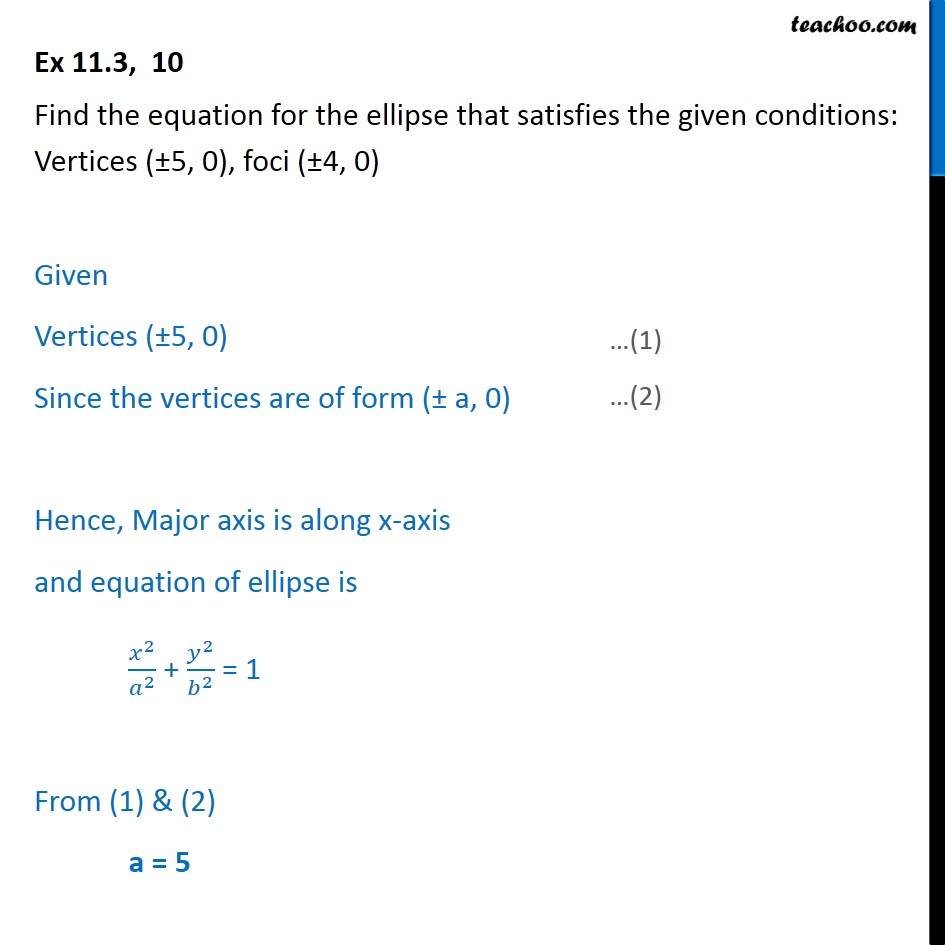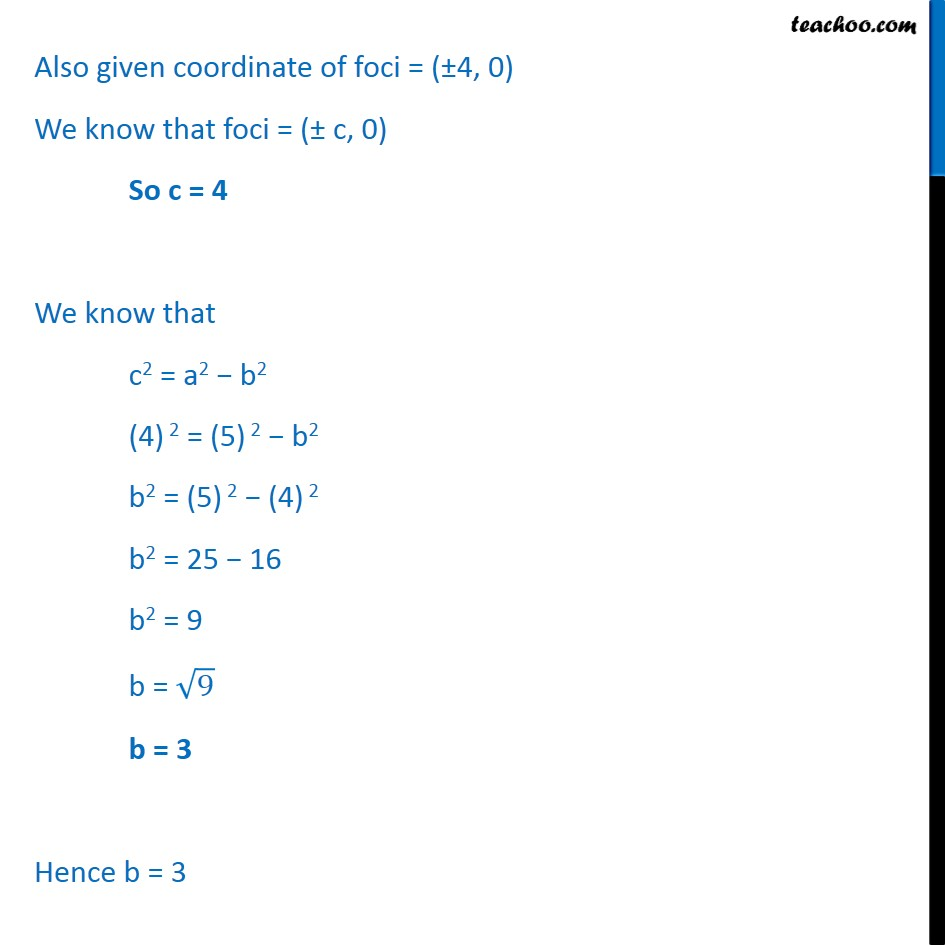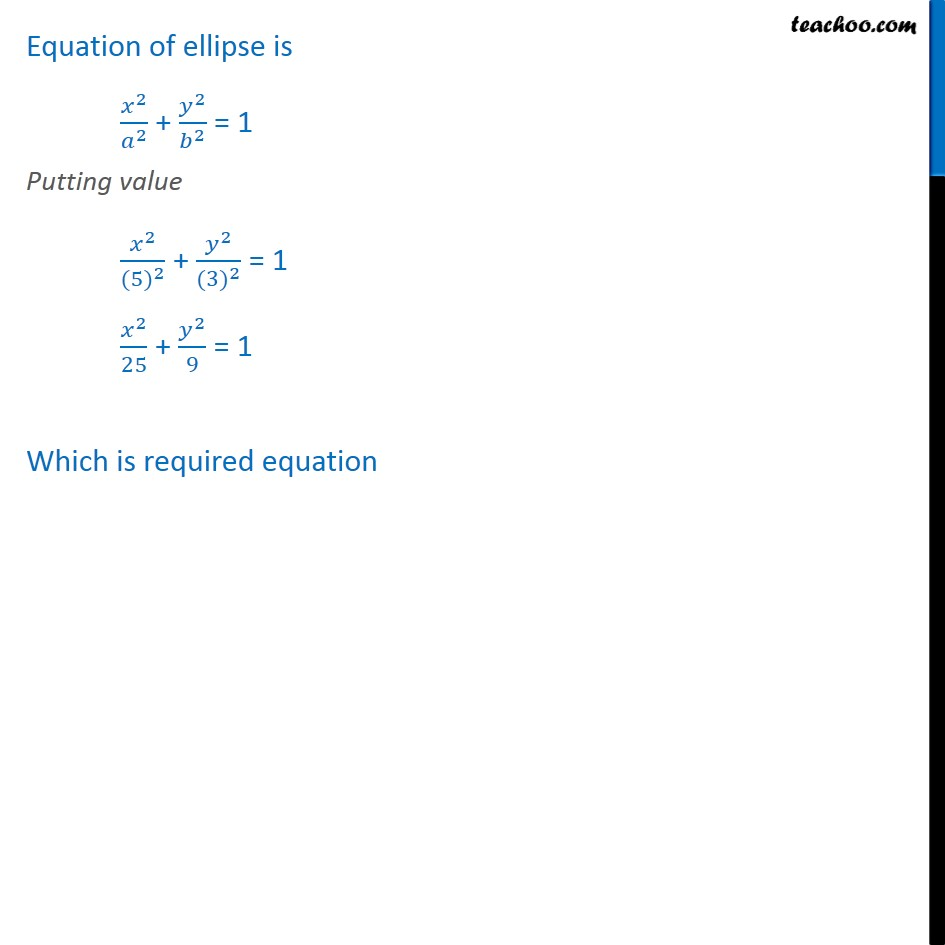Ex 10.3

Chapter 10 Class 11 Conic Sections
Serial order wiseLearn in your speed, with individual attention - Teachoo Maths 1-on-1 Class

### Transcript

Ex 10.3, 10 Find the equation for the ellipse that satisfies the given conditions: Vertices (±5, 0), foci (±4, 0) Given Vertices (±5, 0) Since the vertices are of form (± a, 0) Hence, Major axis is along x-axis and equation of ellipse is ﷐﷐𝑥﷮2﷯﷮﷐𝑎﷮2﷯﷯ + ﷐﷐𝑦﷮2﷯﷮﷐𝑏﷮2﷯﷯ = 1 From (1) & (2) a = 5 Also given coordinate of foci = (±4, 0) We know that foci = (± c, 0) So c = 4 We know that c2 = a2 − b2 (4) 2 = (5) 2 − b2 b2 = (5) 2 − (4) 2 b2 = 25 − 16 b2 = 9 b = ﷐﷮9﷯ b = 3 Hence b = 3 Equation of ellipse is ﷐﷐𝑥﷮2﷯﷮﷐𝑎﷮2﷯﷯ + ﷐﷐𝑦﷮2﷯﷮﷐𝑏﷮2﷯﷯ = 1 Putting value ﷐﷐𝑥﷮2﷯﷮﷐(5)﷮2﷯﷯ + ﷐﷐𝑦﷮2﷯﷮﷐(3)﷮2﷯﷯ = 1 ﷐﷐𝑥﷮2﷯﷮25﷯ + ﷐﷐𝑦﷮2﷯﷮9﷯ = 1 Which is required equation